Skip to main content

# 2.E: Structure and Reactivity: Acids and Bases, Polar and Nonpolar Molecules (Exercises)

These are homework exercises to accompany Chapter 2 of Vollhardt and Schore's "Organic Chemistry" Textmap.

## 2.9: Rotation in Substituted Ethanes

### Q26

A tray of ice cubes is left out on the counter in a room, and the ice cubes melt. If the room is a closed system, what is the total enthalpy of the system (room + ice)? The entropy of the system? What about the free energy? Is this process thermodynamically favorable? How would the temperature of the ice, now water, and the room compare?

### Q27

(a) Using the following bond strengths, calculate the ∆Ho for each of the reactions.

Bond Average Strength (kcal mol-1)
C-C 83
C=C 146
C-H 99
Cl-Cl 57
H-Cl 102
C-Cl 78

(i) CH3-CH=CH2 + Cl2 → CH3-CHCl-CH2Cl

(ii) CH3-CH=CH2 + Cl2 → CH2Cl-CH=CH2 + HCl

(b) One of these reactions as a ∆So <0 (-35 cal mol-1) and the other has a ∆So=0. Assign the two to the equations, and give your reasoning.

(c) Based on both ∆So and ∆Ho calculate ∆Go for each reaction at 30oC and 800oC. Are the reactions favorable at these temperatures?

### Q28

1. In each of the following equations label the Brønsted acid and base, on both sides.
2. Also, determine which way the equilibrium lies, left or right.
3. From the given pKa of each acid calculate the equilibrium constant, Keq. (pKa H3O+ = -1.7)

1.2.3.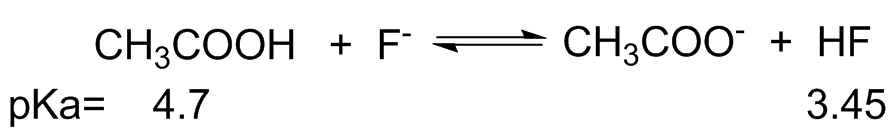4.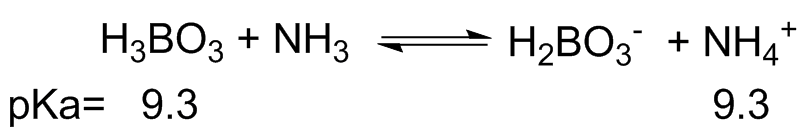### Q29

In problem 28 use electron pushing arrows to show what is occurring, looking from left to right.

### Q30

Determine whether each of the following species is a Lewis acid or Lewis base. Demonstrate this ability with an electron arrow pushing mechanism.

(a) AlCl3 (b) H2O (c) ZnCl2 (d) ScCl3 (e) N3- (f) CH3CH2O-

### Q31

For each of the following molecules denote the bond polarities, δ+ or δ-.

(a)(b)(c)(d)(e)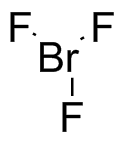### Q32

Identify whether each species is nucleophilic or electrophilic

(a) Fluoride anion, F- (b) Hydride anion, H- (c) acid chloride, CH3COCl (d) ScCl3 (e) N,N'-diethylcarbodiimide### Q33

Identify the functional groups present in each molecule below.

(a)(b)(c)(d)(e)(f)### Q34

Determine, based on Coulombic interaction, if the following are likely to react. No reaction is a possibility.

(a) ammonia (NH3) and cyclohexanone (b) H+ and ethanol (c) azide (N3-) and bromoethane (d) nitrogen (N2) and propane (e) triethylamine and H+

### Q35

Draw the electron arrow pushing mechanism of the potential interaction in problem 34

### Q36

Name each of the following compounds according to IUPAC.

(a)(b)(c)(d)(e)(f)### Q37

Draw the following structures from the name

1. 2,3,4-trimethylpentane
2. 4-ethyl-3,4,5,5-tetramethylnonane
3. neopentane
4. 2,2,4,4-tetramethylpentane
5. 7-pentyltridecane
6. 2,3,4,5,6-pentamethylheptane

### Q38

Draw the structures that correspond to the following names, correct any names that are not in accord with the rules of systematic nomenclature.

1. 3-methyl-2-fluoropentane
2. 1-chloro-3-bromo-2,2-dimethylbutane
3. 2-ethyl-2-chloro-4,5-methylhexane
4. 3-methylpentane

### Q39

Draw all possible isomers of C6H14

### Q40

In each of the following compounds identify each carbon as either primary (1o), secondary (2o), tertiary (3o), or quaternary (4o).

(a) cyclopentane (b) 3,3,5,5-tetraethylheptane (c) methylcyclopentane (d) 2,3,4-trimethylpentane

### Q41

Identify each of the following alkyl groups as primary, secondary, tertiary, or quaternary. This is based off of the 1 position in the alkyl group, connected to the dashed bond. Also, give its IUPAC name.

(a)(b)(c)(d)### Q42

Does the molecule in (a) contain a tertiary carbon? Does the molecule in (b)? Explain why.

(a)(b)### Q43

Based on the following structures, think about the London dispersion forces and how that would affect the boiling point. Rank the following compounds in order from lowest to highest boiling point.

(a) 2,3,4-trimethylhexane (b) nonane (c) 2,3-dimethylpentane (d) 3-methylheptane

### Q44

Draw the lowest energy Newman projection of the following:

1. 2,3-dimethylbutane C2-C3
2. 2,2-dimethylbutane C2-C3
3. 2-methylpentane C2-C3
4. 2-methylpropane

### Q45

Draw the energy diagram, Energy vs. Torsional angle of the C2-C3 bond of butane, rotating increments of 60o starting at the lowest energy conformation. Also draw the Newman projection of each.

### Q46

Given that 85% of all molecules are anti- and 15% of all molecules are eclipsed conformation along a certain C-C bond, take for example C2-C3 of butane, draw the two Newman projections. Calculate the ΔGo value using ΔGo=-RTln(K), at 25oC where R=1.986 cal deg-1 mol-1. Does the given information make sense? If not, in what ways?

### Q47

Identify the functional groups in each compound.

(a)(aspirin) (b)(dopamine) (c)(erythritol- sweetener)

(d)(limonene- in oranges and lemons, subtle differences in stereochemistry make a difference in the smell)

### Q48

Name the alkyl group as if the dashed line were where it was connect to the rest of the molecule, state whether it is primary, secondary, or tertiary.

(a)(b)(c)(d)### Q49

Calculate the new value of k, relative to the initial value, using the Arrhenius equation with increases of 10, 25, and 60oC. Assume the initial temperature is 300oC.

Where Ea is the following

(a) 10 kcal mol-1 (b) 25 kcal mol-1 (c) 50 kcal mol-1

### Q50

An alternate form of the Arrhenius equation involves taking the natural logarithm of the equation giving the following.

lnk=lnA-(Ea/RT)

What plot involving both k and T would yield a straight line? Give some characteristics of the line, slope and intercept.

### Q51

For question 35 draw the product that would result from the arrow pushing mechanism. Make sure not to draw a pentavalent carbon in (a) (use the oxygen atom) and (b) (substitute the bromine and have it leave).

### Q52

Given that the Gibbs free energy is given by the following equation ΔG=ΔH-TΔS, fill in the table that yield a spontaneous process (ΔG<0)

ΔH

ΔS

Less than Zero ???
??? Greater than Zero

### Q53

Under certain conditions the two following reactions are second order, proportional to both reactants concentrations. Write the rate law for each reaction, and similar to in problem 35 draw the structures with any electrostatic interactions.

(a)(b)### Q54

Which of the following is true about 2,3-dimethylbutane?

1. There are 10 primary H's
2. There are 6 times as many primary H's as there are tertiary H's
3. There are 3 tertiary C's
4. There are no primary C's

### Q55

Using ΔG=ΔH-TΔS, where ΔH=-1000 cal/mol and ΔS=-2cal/mol at T=200K, which of the following is true.

1. The reaction is endothermic
2. The reaction is not spontaneous
3. The reaction is exergonic
4. It is likely that the reaction involves one reactant and yields two products.

### Q56

In 2-methylbutane what bond angles exist?

1. 90o
2. 120o
3. 180o
4. 109.5o

### Q57

Which conformation describes the following Newman projection? Which one would be the lowest in energy?1. anti eclipsed
2. anti gauche
3. gauche staggered
4. anti staggered

### Q58

The following molecule, isatin, has all of the following functional groups except for:1. aromatic ring
2. ketone
3. aldehyde
4. amide

### S26

The enthalpy of the system is zero, since the change is cancelled out by the ice gaining heat and the room losing heat. However, the entropy does increase and so the free energy decreases. This process is thermodynamically favorable as the free energy decreases. The end temperature of the ice and water would be equal.

### S27

(a) ∆Ho= Bonds Broken - Bonds Formed

(i) ∆Ho= (C=C + Cl-Cl) - (2 C-Cl + C-C)

= (146 + 57) - (2(78) + 83) = -36 kcal mol-1

(ii) ∆Ho= (C-H + Cl-Cl) - (C-Cl + H-Cl)

=(99 + 57) - (78 + 102) = -24 kcal mol-1

(b) Reaction (i) involves the combination of two molecules into one. Fewer numbers of products concentrates the energy, and therefor leads to a negative ∆So value. Reaction (ii) starts with two molecules and results in two molecules. There is no change in the entropy, and so ∆So=0.

(c) The two above values can be used to find the change in the Gibbs Free Energy using the following equation.

∆Go= ∆Ho - T∆So

(i) at 30oC, or (30 + 273.15)=303.15 K

∆Go= -36 - 303.15(-35x10-3) = -25 kcal mol-1 Favorable

at 800oC, or 973.15 K

∆Go= -36 - 1073.15(-35x10-3) = +1 kcal mol-1 Not Favorable

(ii) at 30oC

∆Go= -24 - 303.15(0) = -24 kcal mol-1 Favorable

at 700oC

∆Go= -24 - 1073.15(0) = -24 kcal mol-1 Favorable

### S28

(a) (i) H2O=Weaker Base HF= Weaker Acid; H3O+= Stronger Acid F-=Stronger Base

(ii) From above, the equilibrium would lie to the left

(iii) Using the pKa we can find the Ka for each acid, Ka=10-pKa HF Ka=10-3.45 and H3O+ Ka= 101.7

Ka HF= [F-][H+]/[HF] and Ka H3O+ =[H+][H2O]/[H3O+] and Keq= [H3O+][F-]/[H2O][HF], from this we can see that Keq= Ka HF/Ka H3O+ or 10-3.45/101.7= 7.08x10-6. This makes sense because the favors the left, so calculating the equilibrium to the right would mean a very small equilibrium constant.

(b) (i) H2O=Weaker Base HCN=Weaker Acid; H3O+=Stronger Acid CN-=Stronger Base

(ii) From part (i), the equilibrium would lie to the left.

(iii) Ka H3O+= [H+][H2O]/[H3O+]= 101.7 and Ka HCN= [CN-][H+]/[HCN]= 10-9.2 and the Keq= Ka HCN/Ka H3O+ = 10-9.2/101.7 = 1.26x10-11 , the case is the same as the previous problem. The equilbrium constant for the reaction as drawn, left to right, is very small.

(c) (i) CH3COOH=Weaker Acid F-=Weaker Base; CH3COO-=Stronger Base HF=Stronger Acid

(ii) Due to part (i), the equilibrium would lie to the left.

(iii) Ka CH3COOH= [H+][CH3COO-]/[CH3COOH]=10-4.7 KHF= [H+][F-]/[HF]=10-3.45 This results in Keq=Ka CH3COOH/KHF= 10-4.7/10-3.45 =5.62x10-2

(d) (i) H3BO3= Equally Acid NH3=Equally Basic; H2BO3-=Equally Basic NH4+=Equally Acidic

(ii) The reaction equilibrium does not lie to either side. H3BO3 and NH4+ are equally acidic according to their pKa

(iii) If the Keq were calculated it be equal to 1. 10-9.3/10-9.3=1.

### S29

(a)(b)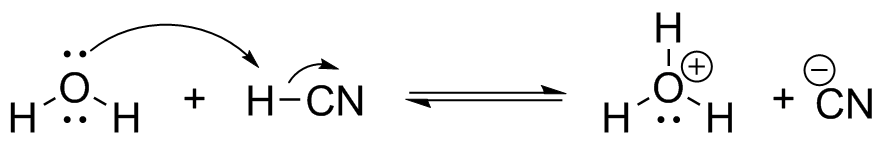(c)(c)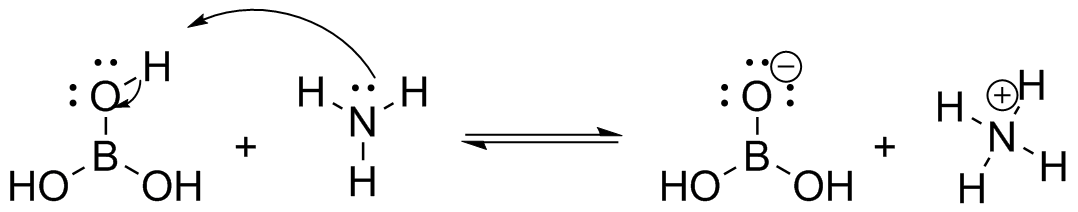### S30

(a) AlCl3 is a Lewis acid. Aluminum is electron deficient, and with three electronegative halogens attached, the Aluminum center is an electron acceptor.(b) H2O is a Lewis base. The oxygen has two lone pairs of electrons, and is thus an electron donor.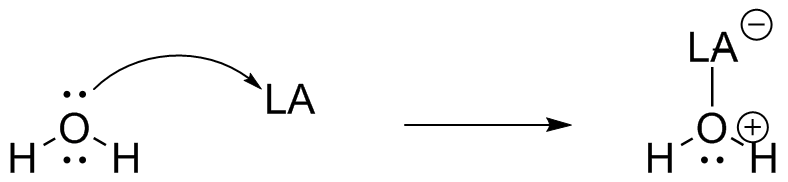(c) ZnCl2 is a Lewis acid. The zinc center is Zn2+, losing two electrons from the 4s orbitals leaving it with a full 3d orbital. It is in a positive oxidation state and has two electronegative halogens attached, and so the zinc center is an electron acceptor.(d) ScCl3 is a Lewis acid. The scandium center is Sc3+, lacking two electrons in the 4s orbitals and one from the 3d orbital, leaving it with a full 3p orbital. It is in a positive oxidation state hand has three electronegative halogens attached, and so the scandium center is an electron acceptor.(e) N3- is a Lewis base. There are two possible resonance structures for this species, and in both there are lone pair electrons on the front nitrogen. It is an electron donor.for simplicity, only one resonance structure was shown as the Lewis acid/base adduct.

(f) CH3CH2O- is a Lewis base. The oxygen has three lone pairs of electrons, it is an electron donor. Even if it was not negatively charged it would still be a Lewis base, with two lone pairs.### S31

(a)(b)(c)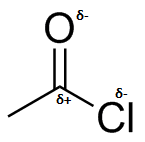(d)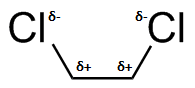(e)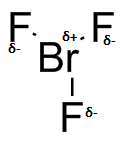### S32

(a) The fluoride anion is negatively charged, and it is nucleophilic. (b) The hydride anion is negatively charged, and it is nucleophilic. (c) Acid chlorides are similar to esters and ketones in that there is an electrophilic carbon double bonded to the oxygen, but acid chlorides are typically much more reactive. (d) ScCl3 was seen earlier to be Lewis acidic, and it is electrophilic. (e) The central carbon in N,N'-diethylcarbodiimide is electrophilic due to the bond polarizations between the two nitrogen atoms.

### S33

(a) An aromatic ring and a carboxylic acid are present. (b) Alcohol (c) ester (d) haloalkane (e) anhydride, this is similar to an ester, but the difference is the oxygen atom placement. (f) amide

### S34

1. The ketone provides an electrophilic carbon.
2. The alcohol has two lone pairs of electrons, and with a positive charge there is a Coulombic attraction.
3. The important thing here is the polarization of the C-Br bond, making the carbon electrophilic. The azide has an overall negative charge, and a Coulombic attraction is present.
4. There is no large polarization in bonds present. Both of these gases are not known for being nucleophilic or electrophilic.
5. This is a standard acid base reaction, but it can be explained by the Coulombic attraction between the positively charged hydrogen ion and the lone pair on the nitrogen.

### S35

(a)(b)(c)(d) No reaction (e)### S36

(a)(b)(c)(d)(e)(f)### S37

(a)(b)(c)(d)(e)(f)### S38

(a)(b)(c)(d)or 2-methylpentane

### S39### S40

(a)(b)(c)(d)### S41

(a) primary, 2,2-dimethylbutyl (b) tertiary, 1-ethyl-1-methylbutyl (c) secondary, 1,3-dimethylbutyl (d) tertiary, 1,1,2,2-tetramethylethyl

### S42

Molecule (a) does not contain a tertiary carbon, it is only bonded to two other carbon atoms. An oxygen atom does not affect this. Compound (b) does contain a tertiary carbon, as it is bonded to three other carbon atoms.

### S43

2,3,4-trimethylpentane<2,3-dimethylhexane<3-methylheptane<nonane

This is a combination of both molecular weight and how branched the molecule is (affect the strength of the London dispersion forces). The higher the molecular weight the higher the boiling point, but also the more branched a molecule is the lower the boiling point.

### S44

(a)(b)(c)(d)### S45### S46ΔGo=-(1.986)(273+25)ln(85/15)= -1027 cal or -1.027 kcal

The given information makes sense in that a larger percentage of the molecules are present in the Anti conformation due to its lower energy. However, other conformations are not included, some lower in energy than the eclipsed form such as staggered. These are likely to also make a contribution.

### S47

(a)(b)(c)(d)### S48

(a) 1,6,6-trimethylhexyl, secondary (b) 1,1,2,2-tetramethylbutyl, tertiary (c) 2,2-dimethylbutyl, primary (d) 2-methylethyl or isopropyl, primary

### S49

The Arrhenius equation is k=Ae-(Ea/RT) where A is a constant. To calculate the new k value use the following k2/k1=Ae-(Ea/RT2)/Ae-(Ea/RT1) and the constant, A, cancels.

k2=[e-(Ea/RT2)/e-(Ea/RT1)]k1 also remember to convert the Ea from kcal to cal.

(a) 10oC - k2=[e-(10,000/(1.986)(310))/e-(10,000/(1.986)(300))]k1= 1.71k1

25oC - k2= 3.64k1

60oC - k2=16.4k1

(b) 10oC - k2=3.87k1

25oC - k2=25.2k1

60oC - k2=1090k1

(c) 10oC- k2=15.0k1

25oC- k2=636k1

60oC- k2=1.19x106k1

Note the increase in affect of the temperature the larger the activation energy.

### S50

lnk=lnA-(Ea/RT)

A plot of lnk vs. 1/T would yield a straight line. The slope of this line would be -(Ea/R) with an x-intercept of Ea/(R(lnA)) and a y-intercept of lnA.

### S51

(a)(b)(c)This is an example of a substitution reaction, which you'll learn about later.

(d) No Reaction (e)### S52

ΔG=ΔH-TΔS

ΔH ΔS
Less than Zero ΔS can either be any positive value, or a negative value such that |TΔS|<|ΔH|
ΔH can either be any negative value, or a positive value such that |ΔH|<|TΔS| Greater than Zero

### S53

(a) Rate=k[CH3CH2O-][alkyl bromide](b) Rate=k[CHC-][alkyl bromide]### S54(b) is correct, there are 12 primary H's and 2 tertiary H's.

(a) There are 10 primary H's - incorrect, there are 12 primary H's

(c) There are 3 tertiary C's - incorrect, there are 2 tertiary C's

(d) There are no primary C's - There are 4 primary C's

### S55

ΔG=ΔH-TΔS=-1000-(200)(-2)=-600 cal/mol, so (c) is true. exergonic means that ΔG<0.

(a) The reaction is endothermic - incorrect, ΔH<0

(b) The reaction is not spontaneous - incorrect, ΔG<0

(d) It is likely that the reaction involves one reactant and yields two products. - incorrect, with a negative change in entropy, it is more likely that two reactants yielded one product.

### S56

This molecule is entirely sp3 hybridized, so all angles are 109.5o, (d).

### S57

(c) - gauche staggered is the conformation. The two CH3 groups are separated by 60o and no group is eclipsed. The lowest energy conformation would be (d) anti staggered, where the larger CH3 groups are anti and there is no eclipsed group.

### S58

(c), aldehyde is not in the molecule. There is a ketone, but no aldehyde.

• Was this article helpful?